# Test: Basics of Digital Electronics

## 10 Questions MCQ Test Topicwise Question Bank for Electronics Engineering | Test: Basics of Digital Electronics

Description
Attempt Test: Basics of Digital Electronics | 10 questions in 30 minutes | Mock test for Electronics and Communication Engineering (ECE) preparation | Free important questions MCQ to study Topicwise Question Bank for Electronics Engineering for Electronics and Communication Engineering (ECE) Exam | Download free PDF with solutions
QUESTION: 1

### The decimal equivalent of (101111.1101)2 is

Solution:

A binary number can be converted into decimal number by multiplying the binary numbers 1 or 0 by their weight and adding the product. Conversion of 101111 is done as follows: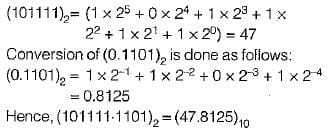QUESTION: 2

Solution:
QUESTION: 3

### Match List-l with List-ll and select the correct answer using the codes given below the lists: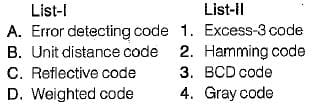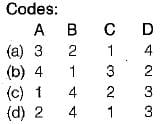Solution:
• “Hamming Code” provides a mathematical way to add one or more parity bits to a data character in order to detect and correct errors.
• “Gray code” is also called a “unit-distance code” because here two adjacent code numbers differ by only one bit.
• “Excess-3 code" is called a “reflective code” because here the code for 9 is the complement of the code for 0, 8 for 1,7 for 2, 6 for 3, and 5 for 4.
• “BCD code” is as “weighted code” or “8421 code” because it uses the binary number system to specify the decimal number 0 to 9.
QUESTION: 4

The following two numbers are converted into desired bases x and y respectively.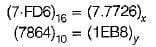The values of x and y are respectively

Solution: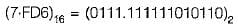The binary number is converted into octal number as follows: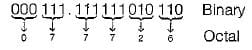Thus,

(7-FD6)16= (7.7726)8

On converting the decimal number (7864)10 into hexadecimal number we obtain the result (EB8)16 as follows: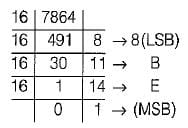Thus, (7864)10 = (1EB8)16

QUESTION: 5

If (100110) x (x)10 = (101010110)2, then the value of x will be equal to

Solution: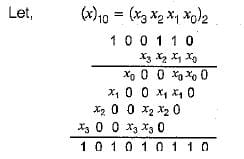From above multiplication, it is clear that,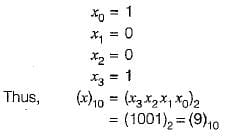QUESTION: 6

The range of numbers that can be represented using 6-bits in signed binary number representation is

Solution:

In signed binary number representation, the range of number that can be represented by an n -bit number is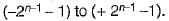For n = 6, the range is -31 to + 31.

QUESTION: 7

A decimal number (22)10 may be represented by the following ways:

Solution:

The binary equivalent of (22)10 = (10110)2 as follows: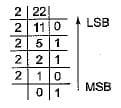∴  (22)10 = (10110)2

• The GRAY code of (10110)2 is obtained as follows: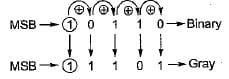Thus, (10110)2 = (11101)gray

• The octal equivalent of (22)10 = (26)8 obtained as follows: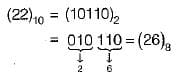• The hexadecimal equivalent of (22)10 obtained as follows: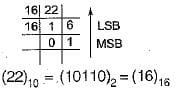QUESTION: 8

The 7-bit even parity Hamming code of the binary bits 0101 is

Solution:

Given,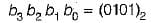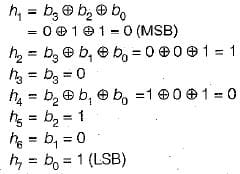∴ The required 7-bit even parity Hamming code is ( 0 1 0 0 1 0 1).

QUESTION: 9

If (2AC9)16 = (Z)7, then the value of Z is

Solution: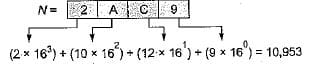Thus, (2AC9)16 - (10,953)10

Now, the decimal number is converted into the number of base 7 as follows: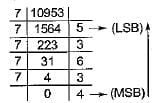Thus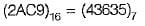QUESTION: 10

Assertion (A): Digital circuits are often called switching circuits.
Reason (R): Each type of digital circuit obeys a certain set of logic rules.

Solution:

Digital circuits are often called switching circuits, because the voltages in a digital circuit are assumed to be switching from one value to another instantaneously, that is, the transition time is assumed to be zero.
Reason is also a correct statement because digital circuit is a logic circuit, because each type of digital circuit obeys a certain set of logic rules. Hence, both assertion and reason are true but reason is not the correct explanation of assertion.Use Code STAYHOME200 and get INR 200 additional OFF Use Coupon Code